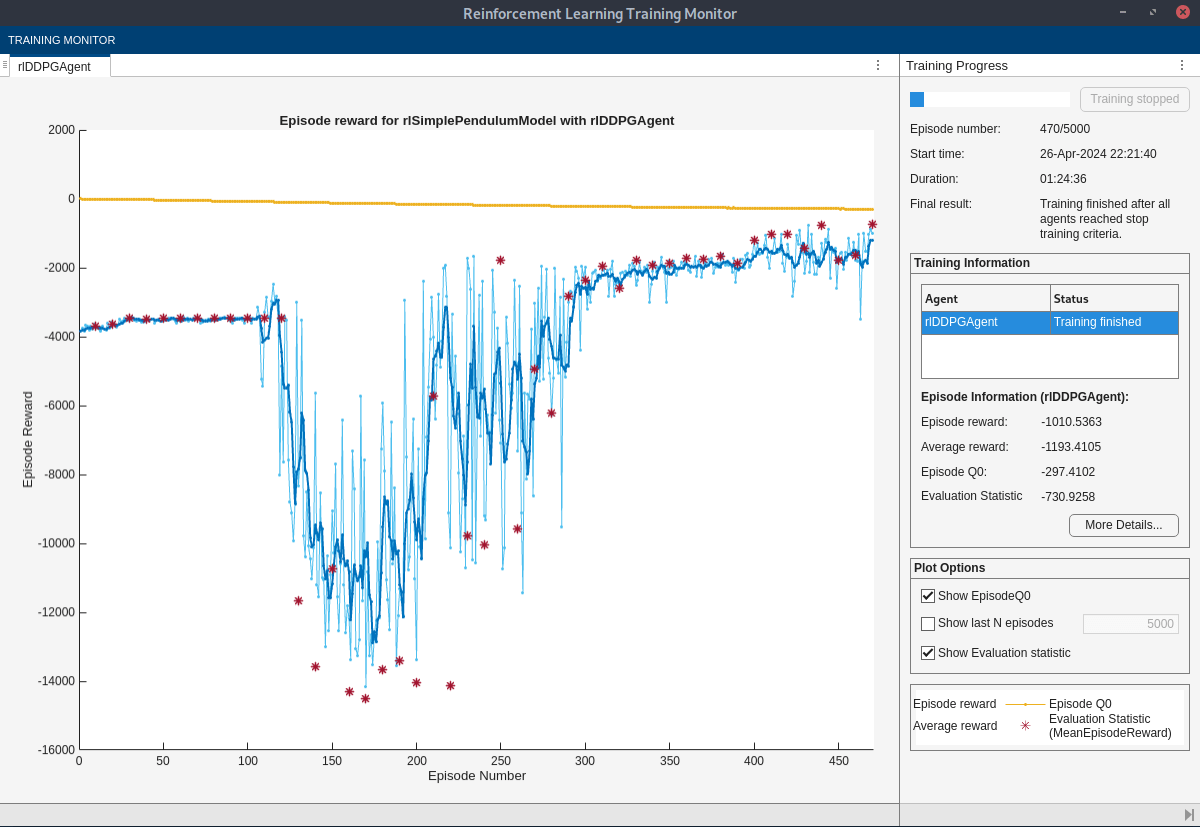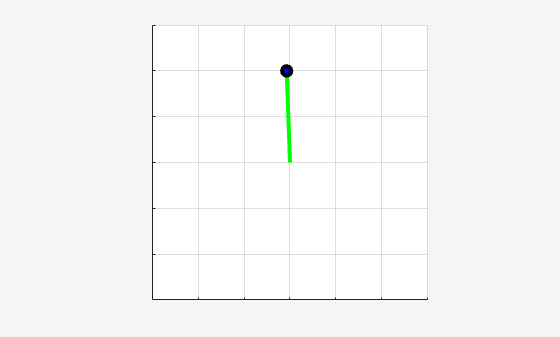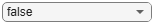# Train DDPG Agent to Swing Up and Balance Pendulum

This example shows how to train a deep deterministic policy gradient (DDPG) agent to swing up and balance a pendulum modeled in Simulink®.

For more information on DDPG agents, see Deep Deterministic Policy Gradient (DDPG) Agents. For an example that trains a DDPG agent in MATLAB®, see Train DDPG Agent to Control Double Integrator System.

### Pendulum Swing-Up Model

The reinforcement learning environment for this example is a simple frictionless pendulum that initially hangs in a downward position. The training goal is to make the pendulum stand upright without falling over using minimal control effort.

Open the model.

```mdl = "rlSimplePendulumModel"; open_system(mdl)```For this model:

• The upward balanced pendulum position is 0 radians, and the downward hanging position is `pi` radians.

• The torque action signal from the agent to the environment is from –2 to 2 N·m.

• The observations from the environment are the sine of the pendulum angle, the cosine of the pendulum angle, and the pendulum angle derivative.

• The reward ${\mathit{r}}_{\mathit{t}}$, provided at every time step, is

`${\mathit{r}}_{\mathit{t}}=-\left({{\theta }_{\mathit{t}}}^{2}+0.1{\stackrel{˙}{{\theta }_{\mathit{t}}}}^{2}+0.001{\mathit{u}}_{\mathit{t}-1}^{2}\right)$`

Here:

• ${\theta }_{\mathit{t}}$ is the angle of displacement from the upright position.

• $\stackrel{˙}{{\theta }_{\mathit{t}}}$ is the derivative of the displacement angle.

• ${\mathit{u}}_{\mathit{t}-1}$ is the control effort from the previous time step.

### Create Environment Interface

Create a predefined environment interface for the pendulum.

`env = rlPredefinedEnv("SimplePendulumModel-Continuous")`
```env = SimulinkEnvWithAgent with properties: Model : rlSimplePendulumModel AgentBlock : rlSimplePendulumModel/RL Agent ResetFcn : [] UseFastRestart : on ```
`obsInfo = getObservationInfo(env);`

The interface has a continuous action space where the agent can apply torque values between –2 to 2 N·m to the pendulum.

`actInfo = getActionInfo(env)`
```actInfo = rlNumericSpec with properties: LowerLimit: -2 UpperLimit: 2 Name: "torque" Description: [0x0 string] Dimension: [1 1] DataType: "double" ```

Set the observations of the environment to be the sine of the pendulum angle, the cosine of the pendulum angle, and the pendulum angle derivative.

```set_param( ... "rlSimplePendulumModel/create observations", ... "ThetaObservationHandling","sincos");```

To define the initial condition of the pendulum as hanging downward, specify an environment reset function using an anonymous function handle. This reset function sets the model workspace variable `theta0` to `pi`.

`env.ResetFcn = @(in)setVariable(in,"theta0",pi,"Workspace",mdl);`

Specify the simulation time `Tf` and the agent sample time `Ts` in seconds.

```Ts = 0.05; Tf = 20;```

Fix the random generator seed for reproducibility.

`rng(0)`

### Create DDPG Agent

DDPG agents use a parametrized Q-value function approximator to estimate the value of the policy. A Q-value function critic takes the current observation and an action as inputs and returns a single scalar as output (the estimated discounted cumulative long-term reward for which receives the action from the state corresponding to the current observation, and following the policy thereafter).

To model the parametrized Q-value function within the critic, use a neural network with two input layers (one for the observation channel, as specified by `obsInfo`, and the other for the action channel, as specified by `actInfo`) and one output layer (which returns the scalar value).

Define each network path as an array of layer objects and assign names to the input and output layers of each path. These names allow you to connect the paths and then later explicitly associate the network input and output layers with the appropriate environment channel.

For more information on creating a deep neural network value function representation, see Create Policies and Value Functions.

```% Define state path statePath = [ featureInputLayer( ... obsInfo.Dimension(1), ... Name="obsPathInputLayer") fullyConnectedLayer(400) reluLayer fullyConnectedLayer(300,Name="spOutLayer") ]; % Define action path actionPath = [ featureInputLayer( ... actInfo.Dimension(1), ... Name="actPathInputLayer") fullyConnectedLayer(300, ... Name="apOutLayer", ... BiasLearnRateFactor=0) ]; % Define common path commonPath = [ additionLayer(2,Name="add") reluLayer fullyConnectedLayer(1) ]; % Create layergraph, add layers and connect them criticNetwork = layerGraph(); criticNetwork = addLayers(criticNetwork,statePath); criticNetwork = addLayers(criticNetwork,actionPath); criticNetwork = addLayers(criticNetwork,commonPath); criticNetwork = connectLayers(criticNetwork,"spOutLayer","add/in1"); criticNetwork = connectLayers(criticNetwork,"apOutLayer","add/in2");```

Convert to `dlnetwork` and display the number of weights.

```criticNetwork = dlnetwork(criticNetwork); summary(criticNetwork)```
``` Initialized: true Number of learnables: 122.8k Inputs: 1 'obsPathInputLayer' 3 features 2 'actPathInputLayer' 1 features ```

View the critic network configuration.

`plot(criticNetwork)`Create the critic approximator object using `criticNet`, the environment observation and action specifications, and the names of the network input layers to be connected with the environment observation and action channels. For more information, see `rlQValueFunction`.

```critic = rlQValueFunction(criticNetwork, ... obsInfo,actInfo, ... ObservationInputNames="obsPathInputLayer", ... ActionInputNames="actPathInputLayer");```

DDPG agents use a parametrized deterministic policy over continuous action spaces, which is implemented by a continuous deterministic actor.

A continuous deterministic actor implements a parametrized deterministic policy for a continuous action space. This actor takes the current observation as input and returns as output an action that is a deterministic function of the observation.

To model the parametrized policy within the actor, use a neural network with one input layer (which receives the content of the environment observation channel, as specified by `obsInfo`) and one output layer (which returns the action to the environment action channel, as specified by `actInfo`).

Define the network as an array of layer objects. Since the output of the hyperbolic tangent layer is always between -1 and 1, use a scaling layer to scale it to the actual range of the action, as specified by `actInfo.UpperLimit`.

```actorNetwork = [ featureInputLayer(obsInfo.Dimension(1)) fullyConnectedLayer(400) reluLayer fullyConnectedLayer(300) reluLayer fullyConnectedLayer(1) tanhLayer scalingLayer(Scale=max(actInfo.UpperLimit)) ];```

Convert to `dlnetwork` and display the number of weights.

```actorNetwork = dlnetwork(actorNetwork); summary(actorNetwork)```
``` Initialized: true Number of learnables: 122.2k Inputs: 1 'input' 3 features ```

Create the actor using `actorNet` and the observation and action specifications. For more information on continuous deterministic actors, see `rlContinuousDeterministicActor`.

`actor = rlContinuousDeterministicActor(actorNetwork,obsInfo,actInfo);`

Specify options for the critic and actor using `rlOptimizerOptions`.

```criticOpts = rlOptimizerOptions(LearnRate=1e-03,GradientThreshold=1); actorOpts = rlOptimizerOptions(LearnRate=1e-04,GradientThreshold=1);```

Specify the DDPG agent options using `rlDDPGAgentOptions`, include the training options for the actor and critic.

```agentOpts = rlDDPGAgentOptions(... SampleTime=Ts,... CriticOptimizerOptions=criticOpts,... ActorOptimizerOptions=actorOpts,... ExperienceBufferLength=1e6,... DiscountFactor=0.99,... MiniBatchSize=128);```

You can also modify the agent options using dot notation.

```agentOpts.NoiseOptions.Variance = 0.6; agentOpts.NoiseOptions.VarianceDecayRate = 1e-5;```

Alternatively, you can create the agent first, and then access its option object and modify the options using dot notation.

Create the DDPG agent using the specified actor, critic, and agent options objects. For more information, see `rlDDPGAgent`.

`agent = rlDDPGAgent(actor,critic,agentOpts);`

### Train Agent

To train the agent, first specify the training options. For this example, use the following options.

• Run training for at most 5000 episodes, with each episode lasting at most `ceil(Tf/Ts)` time steps.

• Display the training progress in the Episode Manager dialog box (set the `Plots` option) and disable the command line display (set the `Verbose` option to `false`).

• Stop training when the agent receives an average cumulative reward greater than –740 over five consecutive episodes. At this point, the agent can quickly balance the pendulum in the upright position using minimal control effort.

• Save a copy of the agent for each episode where the cumulative reward is greater than –740.

For more information, see `rlTrainingOptions`.

```maxepisodes = 5000; maxsteps = ceil(Tf/Ts); trainOpts = rlTrainingOptions(... MaxEpisodes=maxepisodes,... MaxStepsPerEpisode=maxsteps,... ScoreAveragingWindowLength=5,... Verbose=false,... Plots="training-progress",... StopTrainingCriteria="AverageReward",... StopTrainingValue=-740,... SaveAgentCriteria="EpisodeReward",... SaveAgentValue=-740);```

Train the agent using the `train` function. Training this agent is a computationally intensive process that takes several hours to complete. To save time while running this example, load a pretrained agent by setting `doTraining` to `false`. To train the agent yourself, set `doTraining` to `true`.

```doTraining = false; if doTraining % Train the agent. trainingStats = train(agent,env,trainOpts); else % Load the pretrained agent for the example. load("SimulinkPendulumDDPG.mat","agent") end```### Simulate DDPG Agent

To validate the performance of the trained agent, simulate it within the pendulum environment. For more information on agent simulation, see `rlSimulationOptions` and `sim`.

```simOptions = rlSimulationOptions(MaxSteps=500); experience = sim(env,agent,simOptions);```